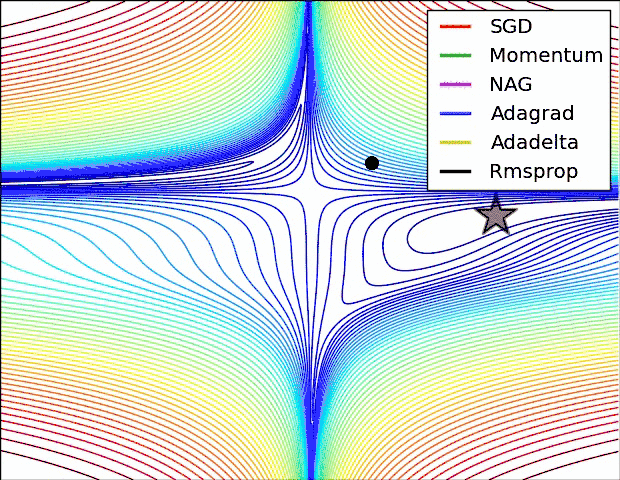# 基本算法

## 动量（momentum）## Nesterov动量

Nesterov动量和标准动量之间的区别体现在梯度计算上。Nesterov动量中，梯度计算在施加当前速度之后。因此，Nesterov动量可以解释为往标准动量方法中添加了一个校正因子。# 自适应学习率的算法

## RMSProp

RMSProp使用指数衰减累积梯度：$r \leftarrow \rho r + (1-\rho) g \odot g$

RMSProp算法还可以和动量结合在一起使用，过程如下：

• 计算临时更新：$\tilde{\theta} \leftarrow \theta + \alpha v$
• 计算梯度：$g \leftarrow \frac{1}{m} \nabla_{\tilde{\theta}} \sum_i L(f(x^{(i)};\tilde{\theta}),y^{(i)})$
• 累积梯度：$r \leftarrow \rho r + (1-\rho) g \odot g$
• 计算速度更新：$v \leftarrow \alpha v -\frac{\epsilon}{\sqrt{r}} \odot g$
• 执行更新：$\theta \leftarrow \theta + v$

AdaDelta算法的作者注意到SGD、Momentum、AdaGrad和RMSProp等算法在参数更新时，单位并不匹配，他认为更新应该和参数应有相同的假想单位，为了实现这一想法，AdaDelta在RMSProp的基础上，还考虑了参数更新的指数衰减累积平方和:$E[\Delta \theta^2]_t = \rho E[\Delta \theta^2]_{t-1} + (1 - \rho) \Delta \theta^2_t$

AdaDelta算法每一步的参数更新为：$\Delta \theta_t = - \dfrac{\sqrt{E[\Delta \theta^2]_{t-1} + \delta}}{\sqrt{E[g^2]_t + \delta}} g_{t}$

Require: 步长 $\epsilon$ （建议默认为： $0.001$）
Require: 矩估计的指数衰减速率， $\rho_1$ 和 $\rho_2$ 在区间 $[0, 1)$内。（建议默认为：分别为$0.9$ 和 $0.999$）
Require: 用于数值稳定的小常数 $\delta$ （建议默认为： $10^{-8}$）
Require: 初始参数 $\theta$
初始化一阶和二阶矩变量 $s = 0$, $r = 0$
初始化时间步 $t=0$
while{没有达到停止准则}
从训练集中采包含$m$个样本$\{ x^{(1)},\dots, x^{(m)}\}$ 的小批量，对应目标为$y^{(i)}$。
计算梯度：$g \leftarrow \frac{1}{m} \nabla_{\theta} \sum_i L(f(x^{(i)};\theta),y^{(i)})$
$t \leftarrow t + 1$
更新有偏一阶矩估计： $s \leftarrow \rho_1 s + (1-\rho_1) g$
更新有偏二阶矩估计：$r \leftarrow \rho_2 r + (1-\rho_2)g \odot g$
修正一阶矩的偏差：$\hat{s} \leftarrow \frac{s}{1-\rho_1^t}$
修正二阶矩的偏差：$\hat{r} \leftarrow \frac{r}{1-\rho_2^t}$
计算更新：$\Delta \theta = - \epsilon \frac{\hat{s}}{\sqrt{\hat{r}} + \delta}$ （逐元素应用操作）
执行更新：$\theta \leftarrow \theta + \Delta \theta$
end while

# 如何选择合适的优化算法# 参考资料

G. Hinton’s lecture

An overview of gradient descent optimization algorithms

Deep Learning book